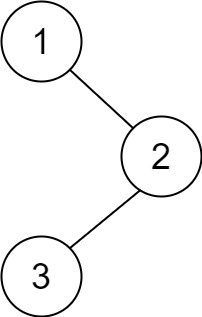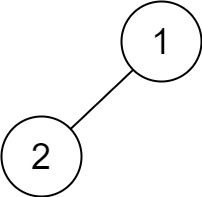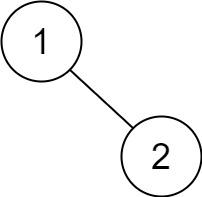# GeetCode Hub

Given the `root` of a binary tree, return the postorder traversal of its nodes' values.

Example 1:```Input: root = [1,null,2,3]
Output: [3,2,1]
```

Example 2:

```Input: root = []
Output: []
```

Example 3:

```Input: root = 
Output: 
```

Example 4:```Input: root = [1,2]
Output: [2,1]
```

Example 5:```Input: root = [1,null,2]
Output: [2,1]
```

Constraints:

• The number of the nodes in the tree is in the range `[0, 100]`.
• `-100 <= Node.val <= 100`

Follow up: Recursive solution is trivial, could you do it iteratively?

/** * Definition for a binary tree node. * public class TreeNode { * int val; * TreeNode left; * TreeNode right; * TreeNode() {} * TreeNode(int val) { this.val = val; } * TreeNode(int val, TreeNode left, TreeNode right) { * this.val = val; * this.left = left; * this.right = right; * } * } */ class Solution { public List<Integer> postorderTraversal(TreeNode root) { } }